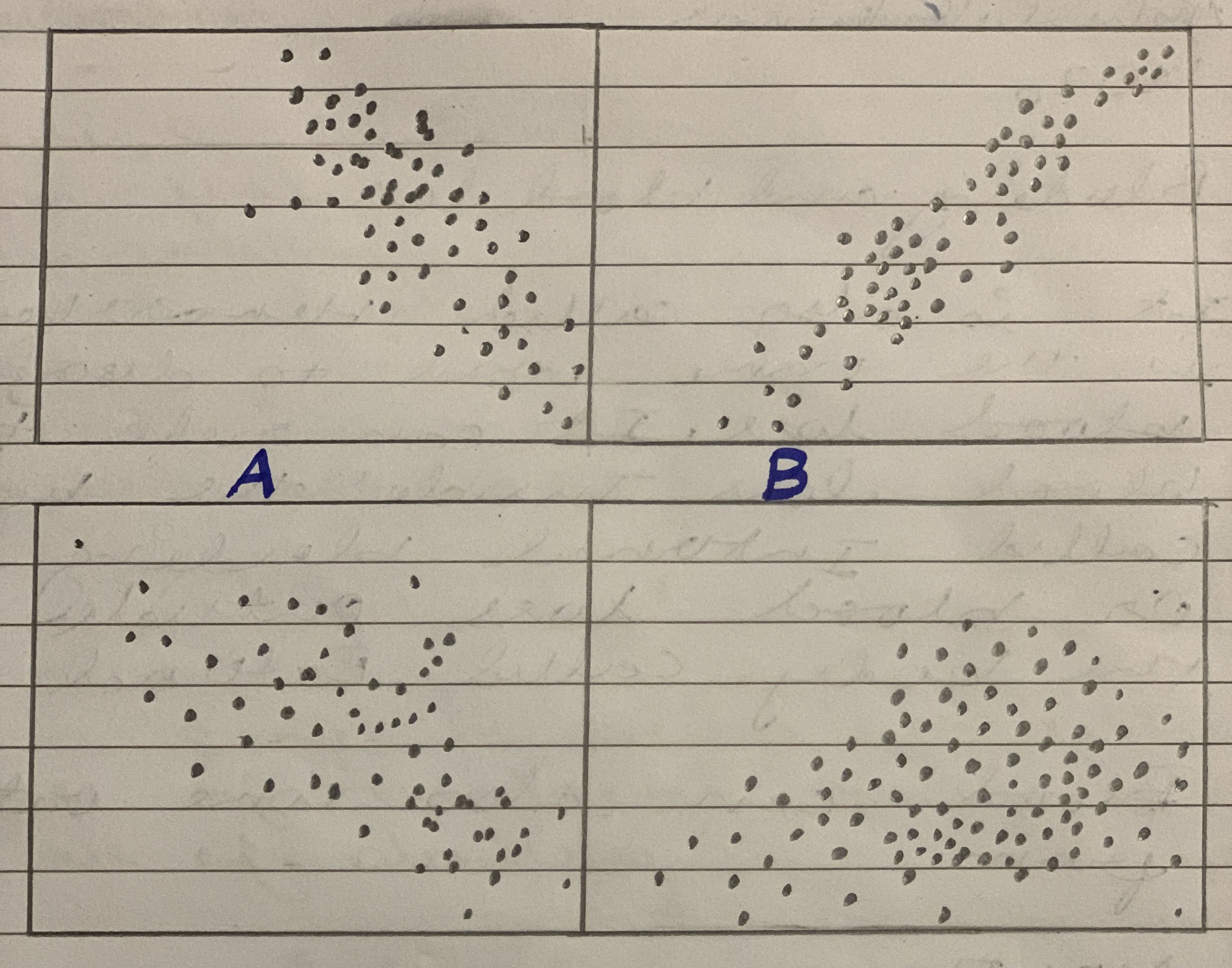# Which two of the four scatter plots have most positive correlation and closer to zero correlation<img src="13310101061.JPG">Wotzdorfg 2020-10-27 Answered

Which two of the four scatter plots have most positive correlation and closer to zero correlationYou can still ask an expert for help

• Questions are typically answered in as fast as 30 minutes

Solve your problem for the price of one coffee

• Math expert for every subject
• Pay only if we can solve itSkladanH
Four scatterplots are given for different data types.
By observe 4 scatter plots, Scatter plot B has increasing values of y when x values are increasing.
Therefore, the scatter plot B has most positive correlation.
Scatter plot C and D has data values randomly. But scatter plot D has most random values compare with scatter plot C.
Therefore, the scatter plot D has closer to zero correlation.